# Archive | December, 2015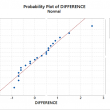## Paired t Test with Minitab

What is a Paired t Test? A Third type of a Two Sample t-Test is the Paired t Test.  This test is used when the two populations are dependent of each other, so each data point from one distribution corresponds to a data point in the other distribution. When using a paired t test, the [...]## Two Sample t Test with Minitab

What is Two Sample T Test? Two sample t test is a hypothesis test to study whether there is a statistically significant difference between the means of two populations. Null Hypothesis (H0): μ1 = μ2 Alternative Hypothesis Ha) : μ1 ≠ μ2 Where: μ1 is the mean of one population and μ2 is the mean of [...]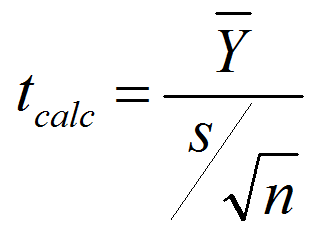## One Sample t Test with Minitab

What is a t Test? In statistics, a t test is a hypothesis test in which the test statistic follows a Student’s t distribution if the null hypothesis is true. We apply a one sample t test when the population variance (σ) is unknown and we use the sample standard deviation (s) instead. A hypothesis [...]## Central Limit Theorem with Minitab

What is Central Limit Theorem? The Central Limit Theorem is one of the fundamental theorems of probability theory. It states a condition under which the mean of a large number of independent and identically-distributed random variables, each of which has a finite mean and variance, would be approximately normally distributed. Let us assume Y1, Y2 [...]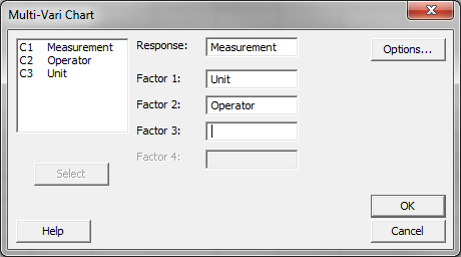## Multi Vari Analysis with Minitab

What is Multi Vari Analysis? Multi Vari analysis is a graphic-driven method to analyze the effects of categorical inputs on a continuous output. It studies how the variation in the output changes across different inputs and helps us quantitatively determine the major source of variability in the output. Multi-Vari charts are used to visualize the [...]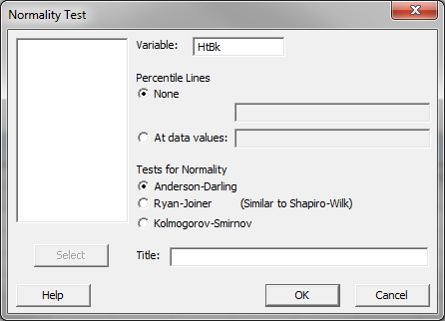## Process Capability with Minitab

What is Process Capability? Process capability measures how well the process performs to meet given specified outcome. It indicates the conformance of a process to meet given requirements or specifications. Capability analysis helps to better understand the performance of the process with respect to meeting customer’s specifications and identify the process improvement opportunities. Process Capability [...]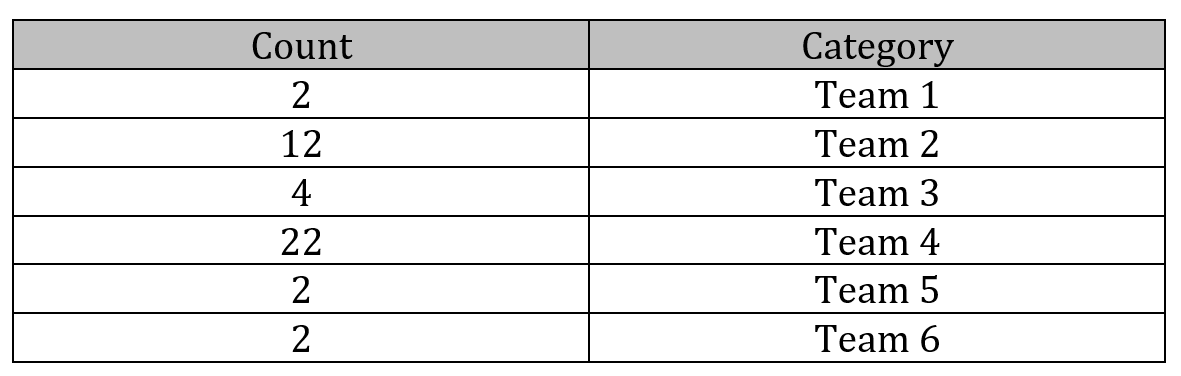## Pareto Analysis with Minitab

What is the Pareto Principle? The Pareto principle is an observation not a law.  Named after Italian economist Vilfredo Pareto, this principle states that for a variety of situations, 80% of consequences come from 20% of the causes, thus the Pareto principle is also known as the 80/20 rule. The 80/20 rule basically reminds us that [...]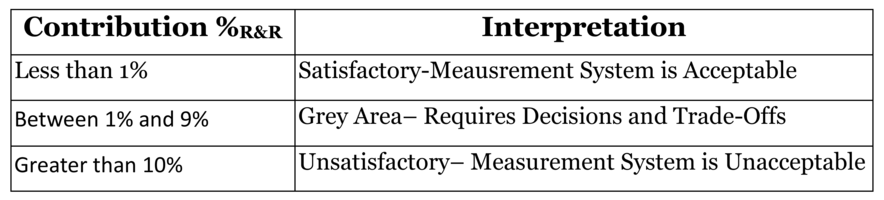## Variable Gage R&R with Minitab

What is Variable Gage R&R? Whenever something is measured repeatedly or by different people or processes, the results of the measurements will vary. Variation comes from two primary sources: Differences between the parts being measured The measurement system We can use a variable Gage R&R to conduct a measurement system analysis to determine what portion [...]## Attribute MSA with Minitab

Use Minitab to Implement an Attribute MSA Data File: “Attribute MSA” tab in “Sample Data.xlsx” (an example in the AIAG MSA Reference Manual, 3rd Edition). Steps in Minitab to run an attribute MSA: Step 1: Reorganize the original data into four new columns (i.e., Appraiser, Assessed Result, Part, and Reference). Click Data → Stack → [...]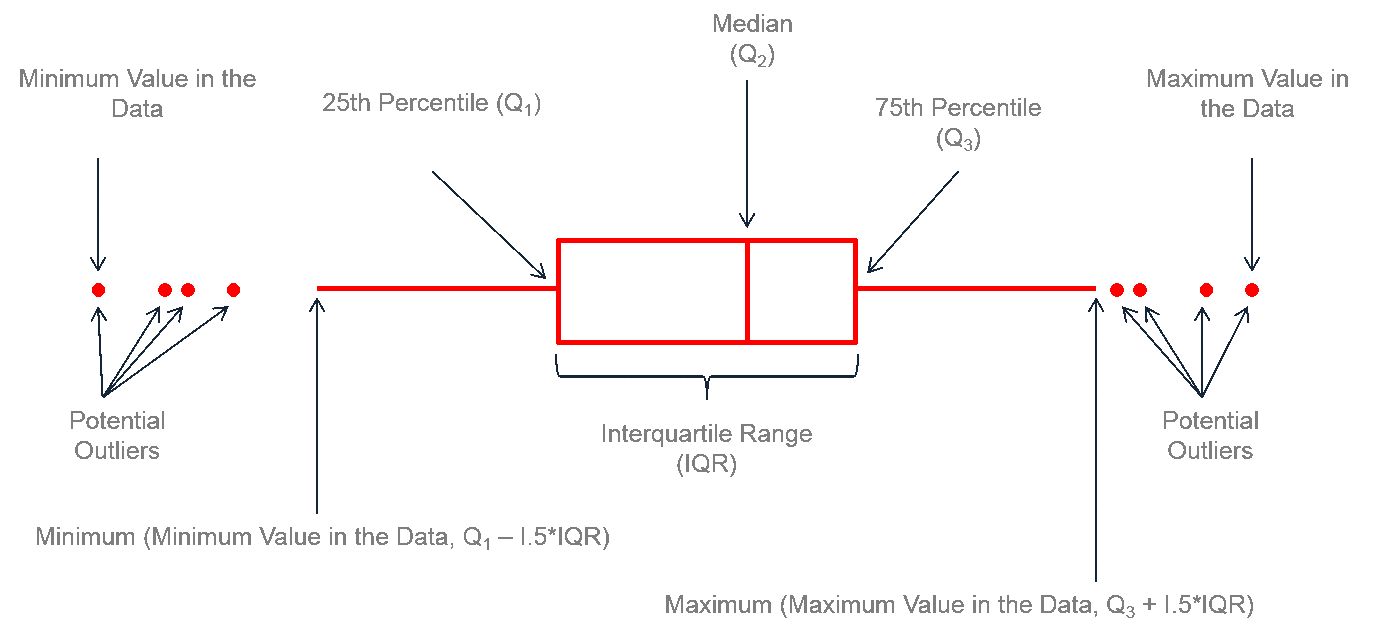## Box Plot with Minitab

What is a Box Plot? A box plot is a graphical method to summarize a data set by visualizing the minimum value, 25th percentile, median, 75th percentile, the maximum value, and potential outliers. A percentile is the value below which a certain percentage of data fall. For example, if 75% of the observations have values [...]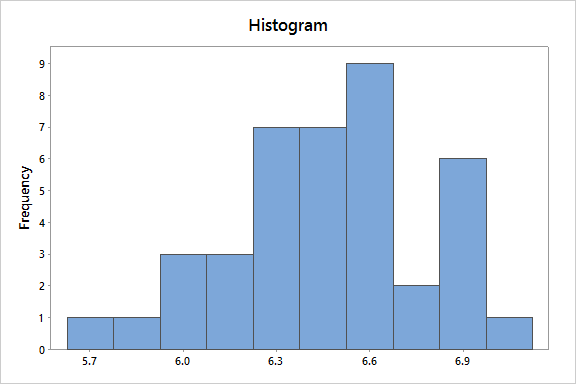## Histogram Rendering with Minitab

What is a Histogram? A histogram is a graphical tool to present the distribution of the data. The X axis represents the possible values of the variable and the Y axis represents the frequency of the value occurring. This graphical summary consists of adjacent rectangles erected over intervals with heights equal to the frequency density of [...]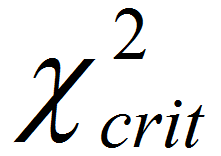## Mood’s Median Test with Sigma XL

What is Mood’s Median Test? Mood’s median test is a statistical test to compare the medians of two or more populations. Null Hypothesis (H0): η1 = … = ηk Alternative Hypothesis (Ha): At least one of the medians is different from the others. The symbol k is the number of groups of our interest and [...]## Kruskal Wallis Test with SigmaXL

Kruskal–Wallis One-Way Analysis of Variance The Kruskal Wallis one-way analysis of variance is a statistical hypothesis test to compare the medians among more than two groups. Null Hypothesis (H0): η1 = η2 = … = ηk Alternative Hypothesis (Ha): at least one of the medians is different from others. Where: ηi is the median of [...]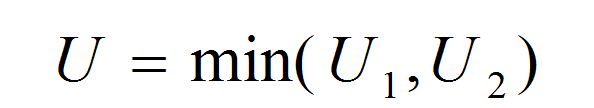## Mann Whitney testing with SigmaXL

What is the Mann Whitney Test? The Mann Whitney test (also called Mann–Whitney U test or Wilcoxon rank-sum test) is a statistical hypothesis test to compare the medians of two populations that are not normally distributed. In a non-normal distribution, the median is the better representation of the center of the distribution. Null Hypothesis (H0): [...]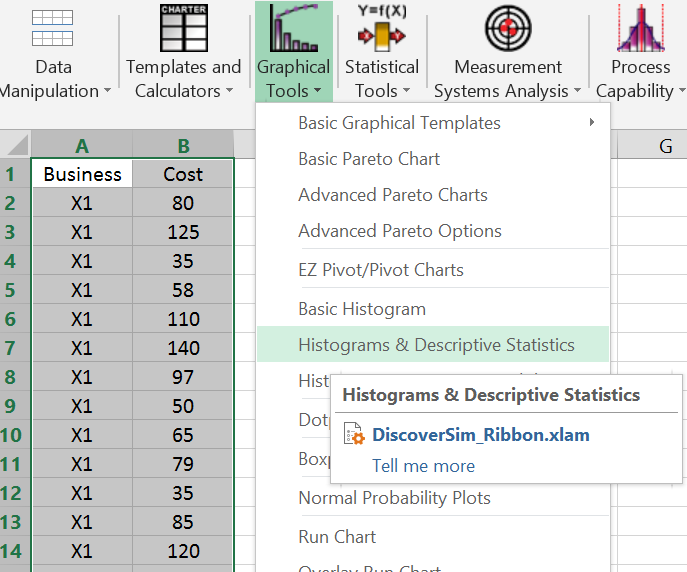## One Way ANOVA with Sigma XL

What is One Way ANOVA? One way Analysis of Variance is a statistical method to compare means of two or more populations. It is a generalized form of the two sample t-test since a two sample t-test compares two population means and one way ANOVA compares k population means where k ≥ 2. Null Hypothesis (H0): μ_1=μ_2=...=μ_k [...]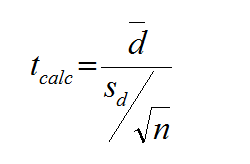## Paired t Test Using SigmaXL

Paired t Test The third type of a Two Sample t-Test is the Paired t Test.  This test is used when the two populations are dependent of each other, so each data point from one distribution corresponds to a data point in the other distribution. When using a paired t test, the test statistic is calculated [...]## Two Sample t Test with SigmaXL

Two Sample t Test Two sample t test is a hypothesis test to study whether there is a statistically significant difference between the means of two populations. Null Hypothesis (H0): μ1 = μ2 Alternative Hypothesis (Ha): μ1 ≠ μ2 Where: μ1 is the mean of one population and μ2 is the mean of the other [...]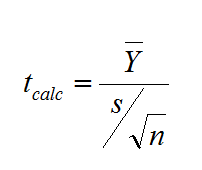## One Sample t Test with SigmaXL

What is a One sample t Test? A One sample t test is a hypothesis test to study whether there is a statistically significant difference between a population mean and a specified value. Null Hypothesis (H0): μ = μ0 Alternative Hypothesis (Ha): μ ≠ μ0 Where: μ is the mean of a population of our [...]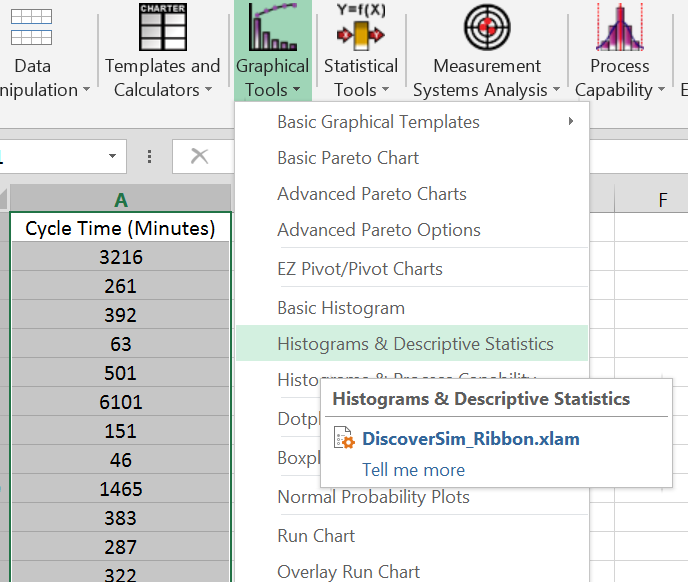## Central Limit Theorem with SigmaXL

Central Limit Theorem The Central Limit Theorem is one of the fundamental theorems of probability theory. It states a condition under which the mean of a large number of independent and identically-distributed random variables, each of which has a finite mean and variance, would be approximately normally distributed. Let us assume Y1, Y2 . . [...]